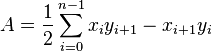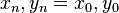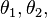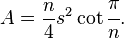# Polygon

In geometry, a polygon is a plane figure that is bounded by a closed path or circuit, composed of a finite sequence of straight line segments (that is, by a closed polygonal chain). These line segments are called its edges or sides, and the points where two edges meet are the polygon's vertices or corners. The interior of the polygon is called its body. A polygon is a 2-dimensional example of the more general "polytope" in any number of dimensions.

In the computer graphics (image generation) field, the term polygon has taken on a slightly altered meaning, more related to the way the shape is stored and manipulated within the computer.

## Classification

### Number of sides

Polygons are primarily classified by the number of sides, see naming polygons below.

### Convexity

Polygons may be characterized by their degree of convexity:

• Convex: any line drawn through the polygon (and not tangent to an edge or corner) meets its boundary exactly twice.
• Non-convex: a line may be found which meets its boundary more than twice.
• Simple: the boundary of the polygon does not cross itself. All convex polygons are simple.
• Concave: Non-convex and simple.
• Star-shaped: the whole interior is visible from a single point, without crossing any edge. The polygon must be simple, and may be convex or concave.
• Self-intersecting: the boundary of the polygon crosses itself. Branko Grünbaum calls these coptic, though this term does not seem to be widely used. The term complex is sometimes used in contrast to simple, but this is mistaken: a complex polygon is one which exists in the unitary plane, which comprises two complex dimensions.
• Star polygon: a polygon which self-intersects in a regular way.

### Symmetry

• Equiangular: all its corner angles are equal.
• Cyclic: all corners lie on a single circle.
• Isogonal or vertex-transitive: all corners lie within the same symmetry orbit. The polygon is also cyclic and equiangular.
• Equilateral: all edges are of the same length. (A polygon with 5 or more sides can be equilateral without being convex.) (Williams, 1979, 31-32)
• Isotoxal or edge-transitive: all sides lie within the same symmetry orbit. The polygon is also equilateral.
• Regular. A polygon is regular if it is both cyclic and equilateral.'. A non-convex regular polygon is called a regular star polygon.

### Miscellaneous

• Rectilinear: a polygon whose sides meet at right angles, i.e., all its interior angles are 90 or 270 degrees.
• Monotone with respect to a given line L, if every line orthogonal to L intersects the polygon not more than twice.

## Properties

We will assume Euclidean geometry throughout.

### Angles

• Any polygon, regular or irregular, complex or simple, has as many corners as it has sides.
• Each corner has several angles. The two most important ones are:
• Interior angle - The sum of the interior angles of a simple n-gon is (n−2)π radians or (n−2)180 degrees. This is because any simple n-gon can be considered to be made up of (n−2) triangles, each of which has an angle sum of π radians or 180 degrees. In topology and analysis,
• Exterior angle - Imagine walking around a simple n-gon marked on the floor. The amount you "turn" at a corner is the exterior or external angle. Walking all the way round the polygon, you make one full turn, so the sum of the exterior angles must be 360°. The exterior angle is the supplementary angle to the interior angle, and from this the sum of the interior angles can be easily confirmed.

The reasoning also applies if some interior angles are more than 180°: going clockwise around, it means that one sometime turns left instead of right, which is counted as turning a negative amount. (Thus we consider something like the winding number of the orientation of the sides, where at every vertex the contribution is between -½ and ½ winding.)

The measure of any interior angle of a convex regular n-gon is (n−2)π/n radians or (n−2)180/n degrees. The interior angles of regular star polygons were first studied by Poinsot, in the same paper in which he describes the four regular star polyhedra.

Moving around an n-gon in general, the sum of the exterior angles (the total amount one "turns" at the vertices) can be any integer times 360°, e.g., 720° For a pentagram and 0° For an angular "eight." See also orbit (dynamics).

### Area and centroid

The area of a polygon is the measurement of the 2-dimensional region enclosed by the polygon. For a non-self-intersecting (simple) polygon with$n$ vertices, the area and centroid are given by:$A = \frac{1}{2} \sum_{i = 0}^{n - 1} x_i y_{i + 1} - x_{i + 1} y_i\,$$\bar x = \frac{1}{6 A} \sum_{i = 0}^{n - 1} (x_i + x_{i + 1}) (x_i y_{i + 1} - x_{i + 1} y_i)\,$$\bar y = \frac{1}{6 A} \sum_{i = 0}^{n - 1} (y_i + y_{i + 1}) (x_i y_{i + 1} - x_{i + 1} y_i)\,$

To close the polygon, the first and last vertices are the same, ie$x_n, y_n = x_0, y_0$. The vertices must be ordered clockwise or counterclockwise, if they are ordered clockwise the area will be negative but correct in absolute value.

The formula was described by Meister in 1769 and by Gauss in 1795. It can be verified by dividing the polygon into triangles, but it can also be seen as a special case of Green's theorem.

The area A of a simple polygon can also be computed if the lengths of the sides, a1,a2, …, an and the exterior angles,$\theta_1, \theta_2,$ …,$\theta_n$ are known. The formula is\begin{align}A = \frac12 ( a_1[a_2 \sin(\theta_1) + a_3 \sin(\theta_1 + \theta_2) + \cdots + a_{n-1} \sin(\theta_1 + \theta_2 + \cdots + \theta_{n-2})] \\ {} + a_2[a_3 \sin(\theta_2) + a_4 \sin(\theta_2 + \theta_3) + \cdots + a_{n-1} \sin(\theta_2 + \cdots + \theta_{n-2})] \\ {} + \cdots + a_{n-2}[a_{n-1} \sin(\theta_{n-2})] ). \end{align}

The formula was described by Lopshits in 1963.

If the polygon can be drawn on an equally-spaced grid such that all its vertices are grid points, Pick's theorem gives a simple formula for the polygon's area based on the numbers of interior and boundary grid points.

If any two simple polygons of equal area are given, then the first can be cut into polygonal pieces which can be reassembled to form the second polygon. This is the Bolyai-Gerwien theorem.

For a regular polygon with n sides of length s, the area is given by:$A = \frac{n}{4} s^2 \cot{\cfrac{\pi}{n}}.$

#### Self-intersecting polygons

The area of a self-intersecting polygon can be defined in two different ways, each of which gives a different answer:

• Using the above methods for simple polygons, we discover that particular regions within the polygon may have their area multiplied by a factor which we call the density of the region. For example the central convex pentagon in the centre of a pentagram has density = 2. The two triangular regions of a cross-quadrilateral (like a figure 8) have opposite-signed densities, and adding their areas together can give a total area of zero for the whole figure.
• Considering the enclosed regions as point sets, we can find the area of the enclosed point set. This corresponds to the area of the plane covered by the polygon, or to the area of a simple polygon having the same outline as the self-intersecting one (or, in the case of the cross-quadrilateral, the two simple triangles).

### Degrees of freedom

An n-gon has 2n degrees of freedom, including 2 for position and 1 for rotational orientation, and 1 for over-all size, so 2n-4 for shape. In the case of a line of symmetry the latter reduces to n-2.

Let k≥2. For an nk-gon with k-fold rotational symmetry (Ck), there are 2n-2 degrees of freedom for the shape. With additional mirror-image symmetry (Dk) there are n-1 degrees of freedom.

## Generalizations of polygons

In a broad sense, a polygon is an unbounded sequence or circuit of alternating segments (sides) and angles (corners). The modern mathematical understanding is to describe this structural sequence in terms of an 'abstract' polygon which is a partially-ordered set (poset) of elements. The interior (body) of the polygon is another element, and (for technical reasons) so is the null polytope or nullitope.

Generally, a geometric polygon is a 'realization' of this abstract polygon; this involves some 'mapping' of elements from the abstract to the geometric. Such a polygon does not have to lie in a plane, or have straight sides, or enclose an area, and individual elements can overlap or even coincide. For example a spherical polygon is drawn on the surface of a sphere, and its sides are arcs of great circles. As another example, most polygons are unbounded because they close back on themselves, while apeirogons (infinite polygons) are unbounded because they go on for ever so you can never reach any bounding end point. So when we talk about "polygons" we must be careful to explain what kind we are talking about.

A digon is a closed polygon having two sides and two corners. On the sphere, we can mark two opposing points (like the North and South poles) and join them by half a great circle. Add another arc of a different great circle and you have a digon. Tile the sphere with digons and you have a polyhedron called a hosohedron. Take just one great circle instead, run it all the way round, and add just one "corner" point, and you have a monogon or henagon.

Other realizations of these polygons are possible on other surfaces - but in the Euclidean (flat) plane, their bodies cannot be sensibly realized and we think of them as degenerate.

The idea of a polygon has been generalized in various ways. Here is a short list of some degenerate cases (or special cases, depending on your point of view):

• Digon. Angle of 0° in the Euclidean plane. See remarks above re. on the sphere.
• Angle of 180°: In the plane this gives an apeirogon), on the sphere a dihedron
• A skew polygon does not lie in a flat plane, but zigzags in three (or more) dimensions. The Petrie polygons of the regular polyhedra are classic examples.
• A spherical polygon is a circuit of sides and corners on the surface of a sphere.
• An apeirogon is an infinite sequence of sides and angles, which is not closed but it has no ends because it extends infinitely.
• A complex polygon is a figure analogous to an ordinary polygon, which exists in the unitary plane.

## Naming polygons

The word 'polygon' comes from Late Latin polygōnum (a noun), from Greek polygōnon/polugōnon πολύγωνον, noun use of neuter of polygōnos/polugōnos πολύγωνος (the masculine adjective), meaning "many-angled." Individual polygons are named (and sometimes classified) according to the number of sides, combining a Greek-derived numerical prefix with the suffix -gon, e.g., pentagon, dodecagon. The triangle, quadrilateral, and nonagon are exceptions. For large numbers, mathematicians usually write the numeral itself, e.g., 17-gon. A variable can even be used, usually n-gon. This is useful if the number of sides is used in a formula.

Some special polygons also have their own names; for example, the regular star pentagon is also known as the pentagram.

Polygon names
Name Edges
henagon (or monogon) 1
digon 2
triangle (or trigon) 3
pentagon 5
hexagon 6
heptagon (avoid "septagon" = Latin [sept-] + Greek) 7
octagon 8
enneagon (or nonagon) 9
decagon 10
hendecagon (avoid "undecagon" = Latin [un-] + Greek) 11
dodecagon (avoid "duodecagon" = Latin [duo-] + Greek) 12
tridecagon (or triskaidecagon) 13
pentadecagon (or quindecagon or pentakaidecagon) 15
icosagon 20
No established English name

"hectogon" is the Greek name (see hectometre),
"centagon" is a Latin-Greek hybrid; neither is widely attested.

100
chiliagon 1000
myriagon 10,000
googolgon 10100

To construct the name of a polygon with more than 20 and less than 100 edges, combine the prefixes as follows

Tens and Ones final suffix
-kai- 1 -hena- -gon
20 icosi- 2 -di-
30 triaconta- 3 -tri-
40 tetraconta- 4 -tetra-
50 pentaconta- 5 -penta-
60 hexaconta- 6 -hexa-
70 heptaconta- 7 -hepta-
80 octaconta- 8 -octa-
90 enneaconta- 9 -ennea-

The 'kai' is not always used. Opinions differ on exactly when it should, or need not, be used (see also examples above).

That is, a 42-sided figure would be named as follows:

Tens and Ones final suffix full polygon name
tetraconta- -kai- -di- -gon tetracontakaidigon

and a 50-sided figure

Tens and Ones final suffix full polygon name
pentaconta-   -gon pentacontagon

But beyond enneagons and decagons, professional mathematicians prefer the aforementioned numeral notation (for example, MathWorld has articles on 17-gons and 257-gons).

## Polygons in nature

Numerous regular polygons may be seen in nature. In the world of minerals, crystals often have faces which are triangular, square or hexagonal. Quasicrystals can even have regular pentagons as faces. Another fascinating example of regular polygons occurs when the cooling of lava forms areas of tightly packed hexagonal columns of basalt, which may be seen at the Giant's Causeway in Ireland, or at the Devil's Postpile in California.

The most famous hexagons in nature are found in the animal kingdom. The wax honeycomb made by bees is an array of hexagons used to store honey and pollen, and as a secure place for the larvae to grow. There also exist animals who themselves take the approximate form of regular polygons, or at least have the same symmetry. For example, starfish display the symmetry of a pentagon or, less frequently, the heptagon or other polygons. Other echinoderms, such as sea urchins, sometimes display similar symmetries. Though echinoderms do not exhibit exact radial symmetry, jellyfish and comb jellies do, usually fourfold or eightfold.

Radial symmetry (and other symmetry) is also widely observed in the plant kingdom, particularly amongst flowers, and (to a lesser extent) seeds and fruit, the most common form of such symmetry being pentagonal. A particularly striking example is the Starfruit, a slightly tangy fruit popular in Southeast Asia, whose cross-section is shaped like a pentagonal star.

Moving off the earth into space, early mathematicians doing calculations using Newton's law of gravitation discovered that if two bodies (such as the sun and the earth) are orbiting one another, there exist certain points in space, called Lagrangian points, where a smaller body (such as an asteroid or a space station) will remain in a stable orbit. The sun-earth system has five Lagrangian points. The two most stable are exactly 60 degrees ahead and behind the earth in its orbit; that is, joining the centre of the sun and the earth and one of these stable Lagrangian points forms an equilateral triangle. Astronomers have already found asteroids at these points. It is still debated whether it is practical to keep a space station at the Lagrangian point — although it would never need course corrections, it would have to frequently dodge the asteroids that are already present there. There are already satellites and space observatories at the less stable Lagrangian points.

## Things to do with polygons

• Cut up a piece of paper into polygons, and put them back together as a tangram.
• Join many edge-to-edge as a tiling or tessellation.
• Join several edge-to-edge and fold them all up so there are no gaps, to make a three-dimensional polyhedron.
• Join many edge-to-edge, folding them into a crinkly thing called an infinite polyhedron.
• Use computer-generated polygons to build up a three-dimensional world full of monsters, theme parks, aeroplanes or anything - see Polygons in computer graphics below.

## Polygons in computer graphics

A polygon in a computer graphics (image generation) system is a two-dimensional shape that is modelled and stored within its database. A polygon can be colored, shaded and textured, and its position in the database is defined by the co-ordinates of its vertices (corners).

Naming conventions differ from those of mathematicians:

• A simple polygon does not cross itself.
• A concave polygon is a simple polygon having at least one interior angle greater than 180 deg.
• A complex polygon does cross itself.

Use of Polygons in Real-time imagery. The imaging system calls up the structure of polygons needed for the scene to be created from the database. This is transferred to active memory and finally, to the display system (screen, TV monitors, etc.) so that the scene can be viewed. During this process, the imaging system renders polygons in correct perspective ready for transmission of the processed data to the display system. Although polygons are two dimensional, through the system computer they are placed in a visual scene in the correct three-dimensional orientation so that as the viewing point moves through the scene, it is perceived in 3D.

Morphing. To avoid artificial effects at polygon boundaries where the planes of contiguous polygons are at different angle, so called 'Morphing Algorithms' are used. These blend, soften or smooth the polygon edges so that the scene looks less artificial and more like the real world.

Polygon Count. Since a polygon can have many sides and need many points to define it, in order to compare one imaging system with another, "polygon count" is generally taken as a triangle. A triangle is processed as three points in the x, y, and z axes, needing nine geometrical descriptors. In addition, coding is applied to each polygon for color, brightness, shading, texture, NVG (intensifier or night vision), Infra-Red characteristics and so on. When analyzing the characteristics of a particular imaging system, the exact definition of polygon count should be obtained as it applies to that system.

Meshed Polygons. The number of meshed polygons (`meshed' is like a fish net) can be up to twice that of free-standing unmeshed polygons, particularly if the polygons are contiguous. If a square mesh has n + 1 points (vertices) per side, there are n squared squares in the mesh, or 2n squared triangles since there are two triangles in a square. There are (n+1) 2/2n2 vertices per triangle. Where n is large, this approaches one half. Or, each vertex inside the square mesh connects four edges (lines).

Vertex Count. Because of effects such as the above, a count of Vertices may be more reliable than Polygon count as an indicator of the capability of an imaging system.

Point in polygon test. In computer graphics and computational geometry, it is often necessary to determine whether a given point P = (x0,y0) lies inside a simple polygon given by a sequence of line segments. It is known as the Point in polygon test.